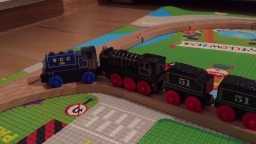# Simultaneously 25481

When and where will two trains that run simultaneously from stations A and B at a distance of 60 km meet if the train from station A ran at 70 km / h and the train from station B at 50 km / h?

t =  0.5 h
s1 =  35 km

### Step-by-step explanation:Did you find an error or inaccuracy? Feel free to write us. Thank you!

Tips for related online calculators
Do you have a linear equation or system of equations and looking for its solution? Or do you have a quadratic equation?
Do you want to convert length units?
Do you want to convert velocity (speed) units?
Do you want to convert time units like minutes to seconds?